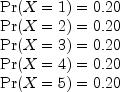### Example 4: Discrete Uniform Random Numbers

In this example, nextDiscrete and nextUniformDiscrete are each used to generate discrete uniform pseudorandom numbers. Note that the probabilities across the possible values must be equal, in order to generate uniformly from nextDiscrete . Also note that since the same random seed is used, the methods produce the same results.import com.imsl.stat.*;
import com.imsl.math.*;

public class RandomEx4 {

static Random IMSLRandom() {
Random r = new Random();
r.setSeed(123457);
r.setMultiplier(16807);
return r;
}

public static void main(String args[]) {
int[] deviates = new int;
int[] deviatesUniform = new int;
double[] prob1 = {.20, .20, .20, .20, .20};
PrintMatrix pm = new PrintMatrix("Random deviates");
PrintMatrixFormat pmf = new PrintMatrixFormat();
Random r = IMSLRandom();

for (int i = 0; i < 5; i++) {
deviates[i] = r.nextDiscrete(1, prob1);
}

pmf.setNoColumnLabels();
pmf.setNoRowLabels();
pm.print(pmf, deviates);

Random rUniform = IMSLRandom();

for (int i = 0; i < 5; i++) {
deviatesUniform[i] = rUniform.nextUniformDiscrete(5);
}

pm.print(pmf, deviatesUniform);
}
}


#### Output

Random deviates

5
2
4
3
5

Random deviates

5
2
4
3
5